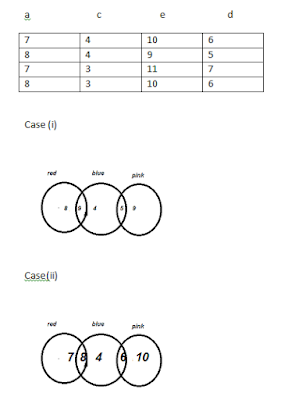# High Level Maths Quiz For SBI POQues 1) Probability of giving right answer by a student is 1/3. If eights questions are asked to a student one after the other, what is the probability that a student gives at least two right answers?

Read the give information carefully and answer the questions ( 2 and 3)
There are 35 employees in an office  49 diaries, each having a pink cover a blue over, or a red cover ,are distributed in a way that employees get at least one diary. The number of employees who got only one diary each with either red or blue or pink cover are 21, of which less than 5 employees got only one diary each with a blue cover, more than 4 employees got only one diary each with a red cover and more than 8 employees got only one diary each with pink cover. The number of employees who got both blue and red covered diaries but not a pink covered one is one more than those who got only red covered diary. Employees who got blue and pink covered diaries but not a red covered one are 4 less than the employees who got only pink covered diary. Nobody got all the three diaries. The number of employees getting only red covered diary is not less than the number of those who got both blue and pink covered diaries but not a red covered one. Nobody got both red and pink diaries.

Ques 2) What is the minimum possible number of red covered diaries.

a) 15
b) 17
c)18
d)19

Ques 3) If the total number of diaries is 52 , find the minimum number of pink covered diaries that would be required.
a) 14
b) 15
c) 16
d) 19

Ques 4) Two candles of the same height are lighted at the same time. The first is consumed in 10 hours and the second in 9 hours. Assuming that each candle burns at its own constant rate, find the number of hours after being lighted , after which the first candle will be twice the height of the second candle.
a) 4 hours
b) 6 hours
c) 8 hours
d) 10 hours

Ques 5) The ratio of Venkat’s salary to Arjun’s salary is 7:4 how much is Arjun’s expenditure ?  (i)The ratio of their savings is 1: 1
(ii) Their expenditure are in the ratio 11:5

a) if only statement (i) alone
b) if only statement (ii)alone
c) if question can be answered using both statement together, but cannot be answered using either alone
d) if using either (i) or (ii) it can be answered
e) if cannot be answered using both statements together.

### Solution 1

Let R be the3 event that the student gives at least two right answers and Rc be the event that he gives at the most one right answer
→ P(R)=1- P( Rc )
( Rc ) is the event that the student gives no right answer or he gives exactly one right answer to the question
→ Let A= event that no right answer is given by the student
→ B = Event that exactly has one right answer is given by the answer
→ P( Rc ) = P(A) + P(B)### Solution 2-3

→ A+b+c+d+e = 35………… (i)
→ A+c+e= 21 ……………… (ii)
→ B+d= 14 ……………..(iii)
→ B=a+1…….. (iv)
→ d=e-4…….. (v)
→ a_> d
→ c<5 ……… (vi)
→ a>4
→ e>8

Using (ii) and (vi), probable values of a,c,e,d are

a   c   e   dAs number of diaries > number of employees , we are sure about number of employees having only red covered, only blue covered or only pink ocered , but the number of employees having 2 dairies might have more than 1 dairy of the same coloured.

Answer 2) using case (ii) the minimum possible number of red covered diaries = 7+8 = 15 diaries

Answer 3) Minimum requirement of pin covered diaries.= 5+ 9 = 14 diaries

### Solution 4

Let the rate of consumption be “10x” metres per hour for first candle
For second candle the rate of consumption be “9y” meters per hour
Since the initial heights are same , we can write
→ 10x= 9y
→ x/y=9/10
Now
Let the candles burn for “t” hours after whicih the first candle will be twice the height fo second candle.the remaining lengths of the two candles can be written as,
→10x-tx = 2(9x-ty)
→ (10-t)x = 2y(9-t)
→ 10-t = 2( )(9-t)
We can substitute
→ x/y= 10-t = 2( )(9-t)
→ 9(10-t) = 2(10)(9-t)
T= 90/11 = 8 hours Answer

### Solution 5

Statement 1 gives the ratio of their savings. If we know the ratio of income as well as savings, we can calculate the ratio of expenditure, but we cannot calculate the absolute values for any of them.
Hence (i) is alone not sufficient.

Statement 2 gives the ratio of expenditure, as no other absolute value is given, only ratio of expenditure is not sufficient to find out the Arjun’s absolute expenditure.
Hence (ii) is alone not sufficient.

Even taking them together you will only get infinite values. This cannot be answered using both.